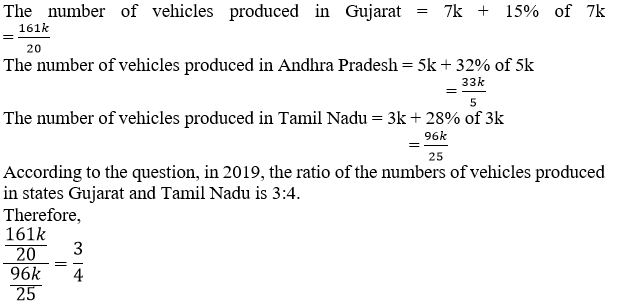# Explanation

In 2018,

Let the number of vehicles produced in state Gujarat = 7k,

Let the number of vehicles produced in state Andhra Pradesh = 5k and

Let the number of vehicles produced in state Tamil Nadu = 3k.

Next year,Now, as we are not given any data related to the ratio of number of vehicles produced in Andhra Pradesh to the number of vehicles produced in either of the two states, therefore, we cannot find the total number of vehicles produced in all the three states and thus, the data is insufficient.

Hence, option c. is correct.